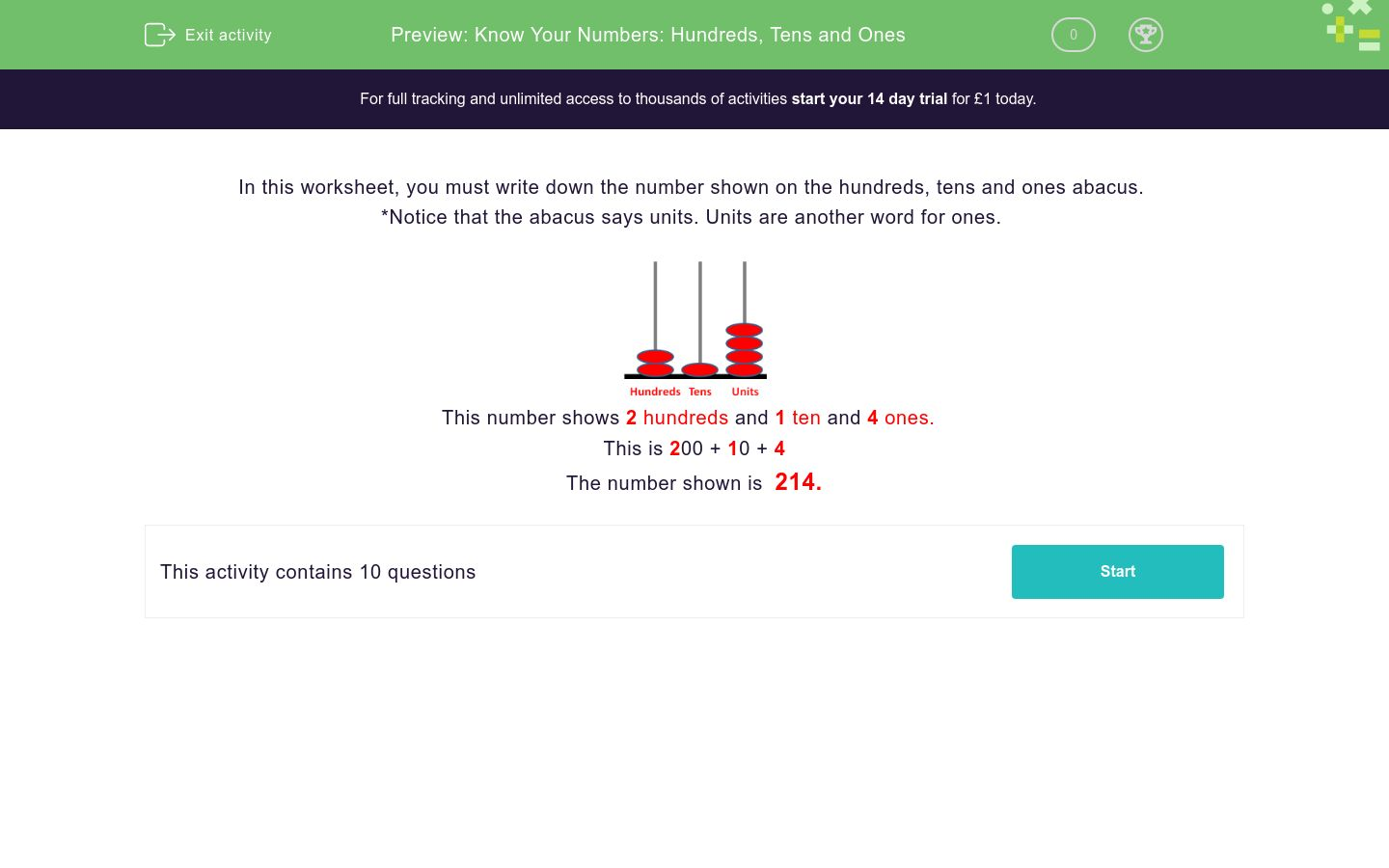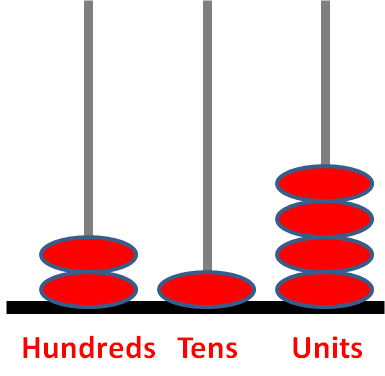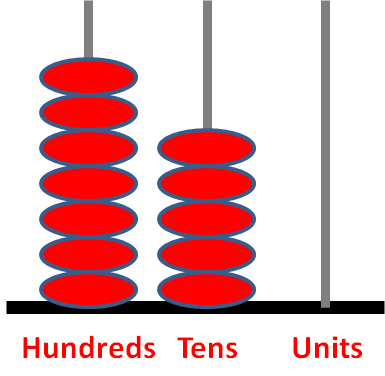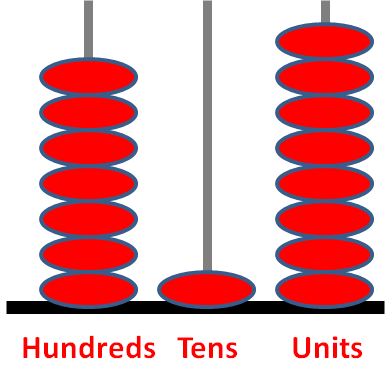# Know Your Numbers: Hundreds, Tens and Ones

In this worksheet, students recognise hundreds, tens and ones. Then they add them together to arrive at a final number.Key stage:  KS 2

Curriculum topic:   Number: Number and Place Value

Curriculum subtopic:   Recognise Hundreds, Tens and Ones

Difficulty level:### QUESTION 1 of 10

In this worksheet, you must write down the number shown on the hundreds, tens and ones abacus.

*Notice that the abacus says units. Units are another word for ones.This number shows 2 hundreds and 1 ten and 4 ones.

This is 200 + 10 + 4

The number shown is  214.

Write down the number shown on the abacus.Write down the number shown on the abacus.Write down the number shown on the abacus.Write down the number shown on the abacus.Write down the number shown on the abacus.Write down the number shown on the abacus.Write down the number shown on the abacus.Write down the number shown on the abacus.Write down the number shown on the abacus.Write down the number shown on the abacus.• Question 1

Write down the number shown on the abacus.213
EDDIE SAYS
200 + 10 + 3 = 213
• Question 2

Write down the number shown on the abacus.623
EDDIE SAYS
600 + 20 + 3 = 623
• Question 3

Write down the number shown on the abacus.971
EDDIE SAYS
900 + 70 + 1 = 971
• Question 4

Write down the number shown on the abacus.750
EDDIE SAYS
700 + 50 + 0 = 750
• Question 5

Write down the number shown on the abacus.718
EDDIE SAYS
700 + 10 + 8 = 718
• Question 6

Write down the number shown on the abacus.718
EDDIE SAYS
700 + 10 + 8 = 718
• Question 7

Write down the number shown on the abacus.645
EDDIE SAYS
600 + 40 + 5 = 645
• Question 8

Write down the number shown on the abacus.864
EDDIE SAYS
800 + 60 + 4 = 864
• Question 9

Write down the number shown on the abacus.768
EDDIE SAYS
700 + 60 + 8 = 768
• Question 10

Write down the number shown on the abacus.605
EDDIE SAYS
600 + 0 + 5 = 605
---- OR ----

Sign up for a £1 trial so you can track and measure your child's progress on this activity.

### What is EdPlace?

We're your National Curriculum aligned online education content provider helping each child succeed in English, maths and science from year 1 to GCSE. With an EdPlace account you’ll be able to track and measure progress, helping each child achieve their best. We build confidence and attainment by personalising each child’s learning at a level that suits them.

Get started